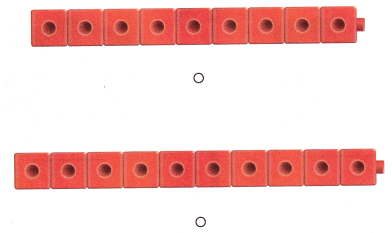# Texas Go Math Kindergarten Lesson 5.3 Answer Key Model, Count and Write 10

Refer to our Texas Go Math Kindergarten Answer Key Pdf to score good marks in the exams. Test yourself by practicing the problems from Texas Go Math Kindergarten Lesson 5.3 Answer Key Model, Count and Write 10.

## Texas Go Math Kindergarten Lesson 5.3 Answer Key Model, Count and Write 10

Explore

DIRECTIONS: Use counters to show 9 in the top ten frame. Use counters to show 10 in the bottom ten frame. Draw the counters.Explanation:
I drew 9 counters in the top ten-frame and 10 counters in the bottom ten-frame to model the numbers 9 and 10.

Share and Show

DIRECTIONS: 1. Count and tell how many eggs. Trace the number. 2-5. Count and tell how many eggs. Write the number.

Question 1.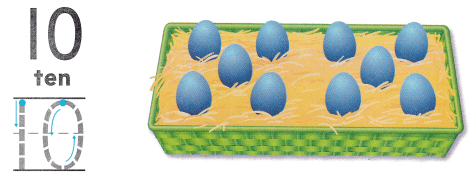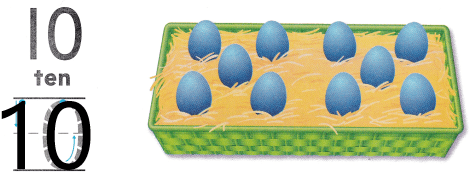Explanation:
I counted and traced the number 10 as there are 10 eggs in the tray.

Question 2.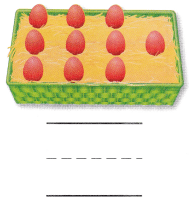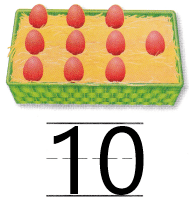Explanation:
I counted and wrote the number 10 as there are 10 eggs in the tray.

Question 3.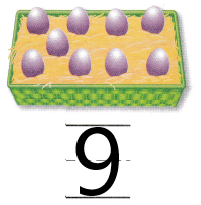Explanation:
I counted and wrote the number 9 as there are 9 eggs in the tray.

Question 4.Explanation:
I counted and wrote the number 10 as there are 10 eggs in the tray.

Question 5.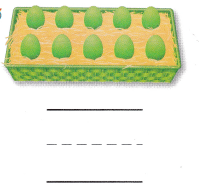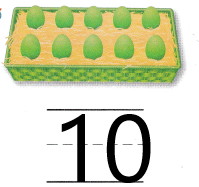Explanation:
I counted and wrote the number 10 as there are 10 eggs in the tray.

DIRECTIONS: 6. Say the number. Trace and write the numbers. 7-9. Count and tell how many. Write the number.

Question 6.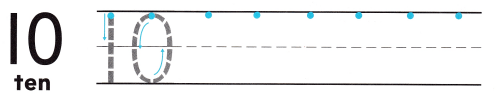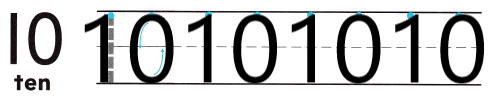Explanation:
I traced and practiced writing the number 10.

Question 7.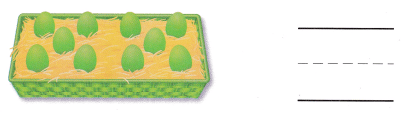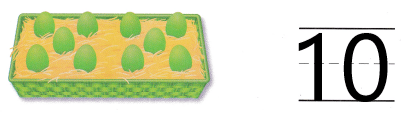Explanation:
I counted and wrote the number 10 as there are 10 eggs in the tray.

Question 8.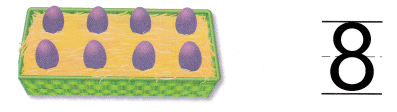Explanation:
I counted and wrote the number 8 as there are 8 eggs in the tray.

Question 9.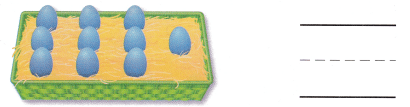Explanation:
I counted and wrote the number 10 as there are 10 eggs in the tray.

DIRECTIONS: 10. Count the stars in each set. Which sets show ten stars? Circle those sets. 11. Choose the correct answer. Ed counts 10 cubes Which set is his?

Problem Solving

Question 10.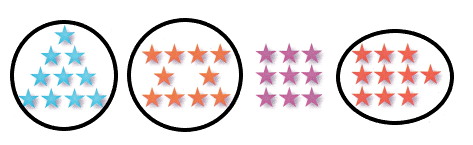Explanation:
i drew circle around the groups of stars that are 10 in number.

Question 11.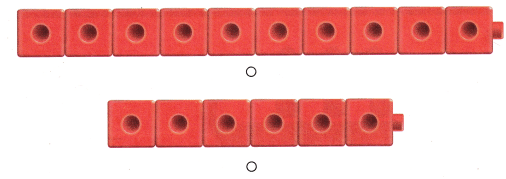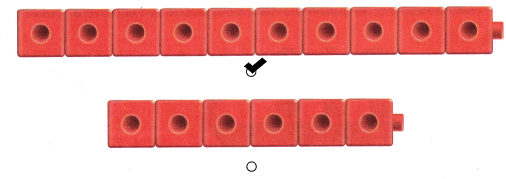Explanation:
I marked the set of cubes that has 10 cubes.

### Texas Go Math Kindergarten Lesson 5.3 Homework and Practice Answer Key

DIRECTIONS: 1-4. Count and tell how many apples. Write the number.

Question 1.Explanation:
I counted and wrote the number 8 as there are 8 apples.

Question 2.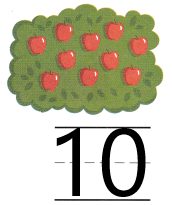Explanation:
I counted and wrote the number 10 as there are 10 apples.

Question 3.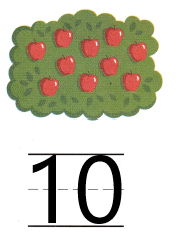Explanation:
I counted and wrote the number 10 as there are 10 apples.

Question 4.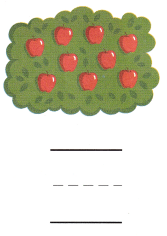Explanation:
I counted and wrote the number 9 as there are 9 apples.

DIRECTIONS: Choose the correct answer. 5. Lesli counts 8 cubes Which set is hers? 6. Lennie counts 10 cubes. Which set is his?

Lesson Check

Question 5.Explanation:
I marked the set of cubes that has 8 cubes.

Question 6.